### Home > A2C > Chapter 12 > Lesson 12.5.1 > Problem12-197

12-197.
1. Prove that the expressions in each of the following pairs are equivalent by showing the algebraic steps needed to rewrite the one on the left so it is the same as the one on the right. Homework Help ✎

1.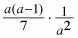,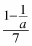2.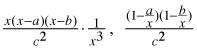3.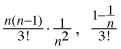4.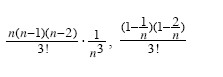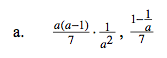Simplify the numerator of the first fraction, then multiply.

$\frac{a^2-a}{7}\cdot\frac{1}{a^2}$

$\frac{1-\frac{1}{a}}{7}$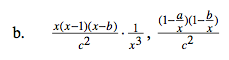$\frac{(1-\frac{a}{x})(1-\frac{b}{x})}{c^2}$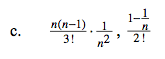See part (a).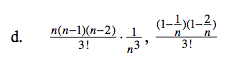See part (b).